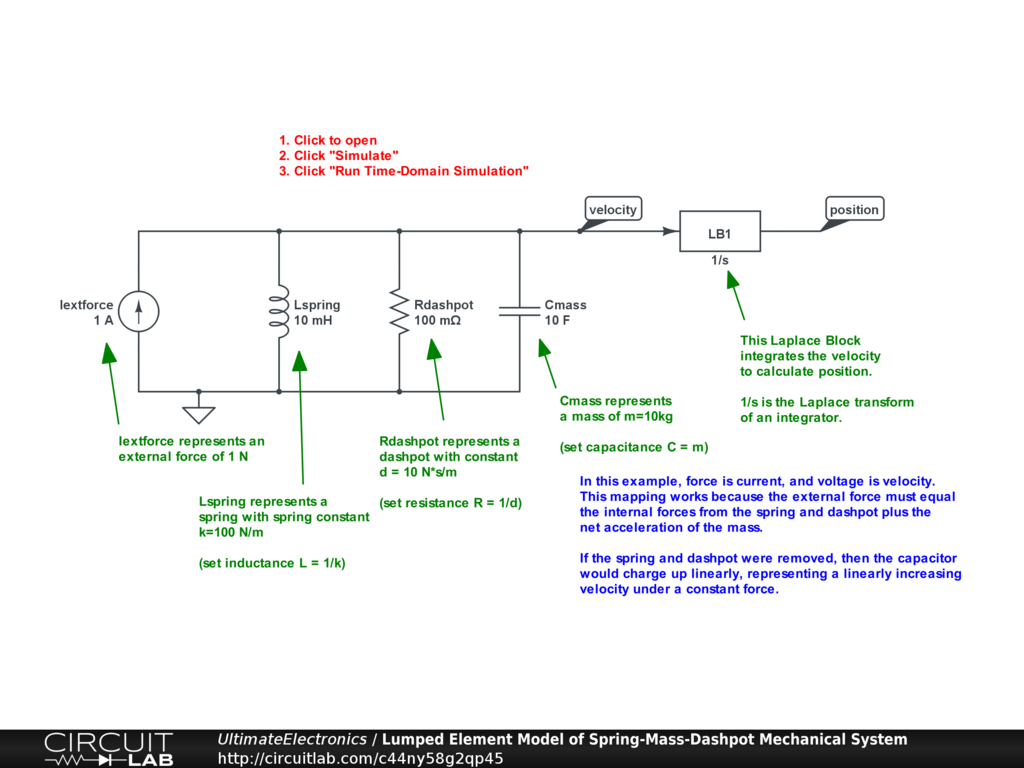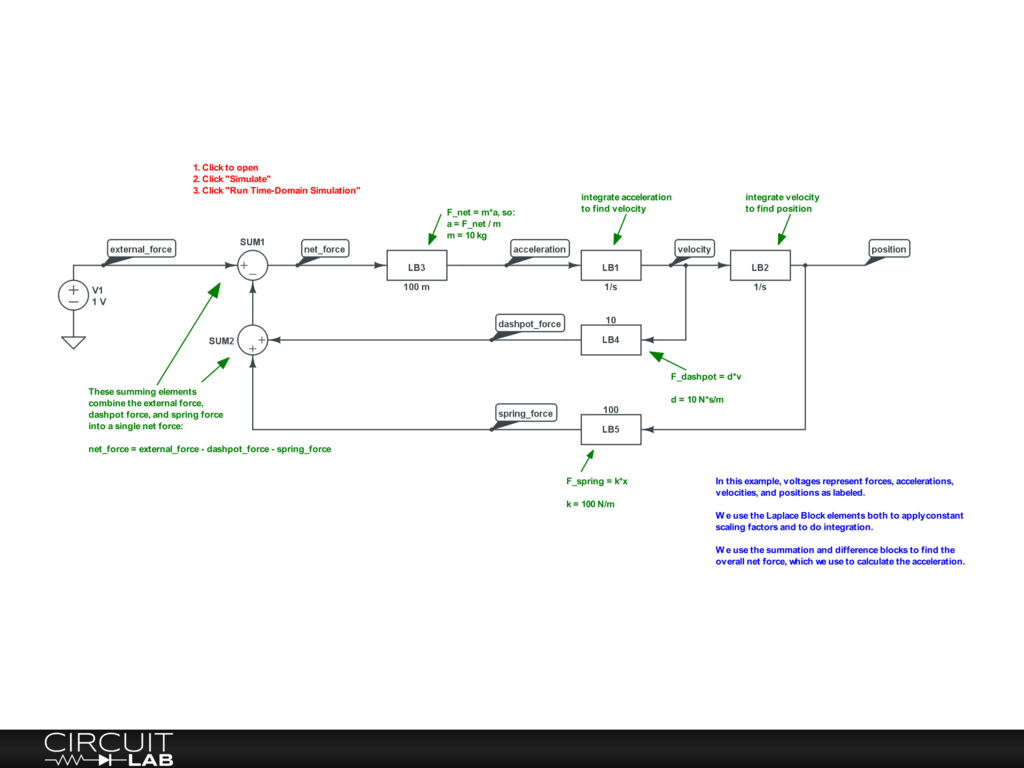Lumped Element Model

In physical reality, a single resistor is a physical system containing (more than) trillions of individual atoms and electrons, with a complicated geometry, grain structure, random thermal motion, and random collisions. Despite that enormous complexity, from an electronics perspective, we treat those trillions of interactions as if they are equivalent to a single $V = I R$ linear equation called Ohm's Law. This is called the lumped element model, and it means we don't have to track the positions of all those individual atoms and electrons and simulate their interactions and behavior. Instead, we can just treat the resistor as one single lumped element and make simplifications that will work with certain ranges of validity.

Just as we discussed in the Steady State & Transient section, the law of large numbers concept from probability and statistics lets us make dramatic macroscale simplifications when we look at the combination of huge numbers of underlying random microscale events.

Describing Complex Systems

In order to describe complex systems, we must:

1. Break down complex systems into discrete chunks with a small number of inputs and outputs.
2. Apply algebraic, numerical, or conceptual abstractions that describe the behavior of that discrete chunk at an aggregate or average level.
3. Understand the limits of those abstractions and approximations.
4. Cascade those chunks together through their inputs and outputs.

The correct model to use for design and analysis problems is the simplest model that covers the phenomenon we're interested in, but no simpler.

A good rule of thumb for any engineering problem is to understand your components at one level of detail deeper than you're actually interested in for your particular design problem. That way, you'll be aware of when you are running up against the limits of your abstractions and approximations.

Lumped Element Model

The lumped element model is a set of assumptions that let us treat various systems as being a single element that behaves like a single entity.

This is much like the idea of a rigid body in elementary mechanics. In physical reality, no solid object is truly rigid under all circumstances, if for no other reason than that the speed of light limits the ability for information about forces at one end of the object to update the motion at the other end of the object instantaneously. While it's fictional, a rigid body is still an extremely useful abstraction for doing statics & dynamics as long as we understand its limitations.

In the same way, the lumped element model lets us, for example condense the interacting behavior of trillions of atoms into a single equation we call a resistor.

In an electronics context, the lumped element model lets us consider:

• We can treat macro-scale objects like a resistor as one or a few equations that relate what happens at its terminals.
• We can treat the object as being physically small enough that it all responds at once as a single unit over our timescales of interest.
• We can treat a node as a single point where two or more elements connect.
• Wires connecting components work instantaneously, have no resistance, capacitance, or inductance (unless explicitly modeled as separate elements).
• Assumptions of how charge & voltage work, which are embodied in Kirchhoff's Voltage Law and Kirchhoff's Current Law, which we'll discuss later.
• In electronics, the lumped element model specifically means that the voltage-to-current relationship of a component is all that's needed to described its behavior. We'll talk more about different circuit elements and their V-I relationships in later sections.

The lumped element model is an approximation, not exact. Still, it's an approximation that enables the rest of the study of electronics, and can also be applied to other systems: thermal, mechanical, and more.

If our lumped element model is too coarse to describe the behavior we're trying to design or analyze, we must break the system down into smaller discrete chunks until we can model the behavior we're interested in. This is similar to the higher-level linearity abstractions we discussed in Linear & Nonlinear, and to the higher-level steady state abstractions we discussed in Steady State & Transient.

Note that as we continue to break down the system into smaller and smaller chunks, the lumped element model becomes quite similar to the finite element model, often used for structural and fluid dynamics calculations.

Mechanical Modeling Example

Another reason the lumped element model is powerful is because it allows for cross-domain modeling. When we treat systems of any kind as having certain macroscale elements with simple rules they obey, we can use convenient electronics simulation software for modeling all kinds of systems.

We can use the lumped element model to figure out what happens to a spring-mass-dashpot mechanical system when an external force is applied. A spring-mass-dashpot system is a good, simplified model for many real elastic systems, such as a car suspension, or structural problems like buildings and bridges. You can also think about it in more natural terms: for example, consider a bird landing on the branch of a tree. How will the branch move as a result of the added external force from the weight of the bird?

Without explaining the modeling details here:

1. The spring keeps the mass connected to the ground. Additionally, the spring alone determines the long-term position of the object after it's reached its new steady state. We map a spring constant $F = k x$ to an inductance $L = \frac {1} {k}$.
2. The mass provides inertia, and force must be applied to accelerate it and decelerate it as it moves. We convert an intertial mass from $F = m a$ to a capacitor $C = m$.
3. The dashpot dissipates energy due to friction or viscous interactions with the surrounding air, for example. Without the dashpot, the spring and mass would keep oscillating forever. We convert a viscous frictional interaction $F = d v$ to a resistance $R = \frac {1} {d}$.

This can be described by a differential equation:

\begin{align} F_{\text{net}} & = F_{\text{spring}} + F_{\text{dashpot}} + F_{\text{external}} \\ m \ddot{x} & = (- k x) + (- d \dot{x}) + F_{\text{external}} \\ m \ddot{x} + d \dot{x} + k x & = F_{\text{external}} \end{align}

This maps to the second-order differential equation describing the voltage of a parallel RLC circuit:

$$C \dot{v} + \frac{1}{R} v + \frac{1}{L} \int v(t) dt = I_{\text{external}}$$

This equation comes from applying Kirchhoff's Current Law to the three parallel paths (capacitor, resistor, inductor) supplied by one current source.

If we map voltage to velocity, then $v = \dot{x}$, and we can finish by mapping $C = m, R = \frac {1} {d}, L = \frac {1} {k}, I_{\text{external}} = F_{\text{external}}$.

This example circuit simulates the unit step response of a system with parameters $m = 10 \ \text{kg}, k = 100 \ \frac{\text{N}} {\text{m}}, d = 10 \ \frac {\text{N s}} {\text{m}}$:Interactive Exercise Click the circuit, click "Simulate," and "Run Time-Domain Simulation." You'll see the motion of the mass plotted when we apply a new external force of $I_{\text{extforce}} = 1 \ \text{N}$ starting at $t=0$.

1. Note that we used the Skip Initial simulation setting to force the simulator to start without finding the steady-state DC solution. See also the Switches section of Chapter 2 for a number of other ways we can impose initial conditions or describe external sources, including using piecewise linear signals, using a CSV file with defined values, and using on-off switches.
2. We used a Laplace Block element set to "1/s" as an integrator to go from velocity to position.

A Laplace Block lets us enter the Laplace Transform of a signal processing element and get the resulting behavior directly as a voltage-in, voltage-out element on our schematic. The Laplace Transform of an integrator is "1/s" so we can use that to quickly construct an ideal voltage integrator. We can also set a Laplace Block to a constant value, for example "2". This would mean that the output voltage would be exactly double the input voltage.

Alternatively, instead of using circuit elements, we could model the entire system using Laplace Blocks. This can make the flow of forces, velocities, and displacements a lot more obvious to follow:Interactive Exercise Click the circuit, click "Simulate," and "Run Time-Domain Simulation." You'll see the same graph of motion as in the earlier example. In this version, however, it's easy to pull out other signals you might be interested in. For example, you can also quickly plot the acceleration curve, which is not so straightforward to find on the circuit model above.

Modeling systems of differential equations with Laplace Blocks and the summation and difference blocks can make the opaque concept of differential equations become much more accessible and concrete.

These models allow for quick testing of various changes to see how they affect the response of the system. On either the circuit element model or the Laplace Block model, try these changes (one at a time!):

1. What happens if we make the dashpot have higher friction? Try setting $d = 60 \ \frac {\text{N s}} {\text{m}}$. How does the shape of the step response change?
2. What if it's a much heavier mass? Try setting $m = 100 \ \text{kg}$. How does the shape of the step response change?
3. What if it's a stiffer spring? Try setting $k = 1000 \ \frac{\text{N}} {\text{m}}$. How does the shape of the step response change?

We can try these parameter changes one at a time and then re-run the simulation to see the results.

However, we can also do a parameter sweep, which asks the simulator to try a bunch of different values for us and then plot all the results on top of each other for easy visualization and comparison. Try this example:Interactive Exercise Click the circuit, click "Simulate," and "Run Time-Domain Simulation." In this case, we've configured the Sweep Parameter simulation setting to adjust the dashpot value. Instead of just simulating the system once for $d=10$, this new setting will simulate it separately for $d=5,10,15,20,\dots,50$, and then show all plots at once. Parameter Sweeps are a fast way to see how one parameter can affect the behavior of a system.

We can use a model like this to adjust not only the parameters of the system, but also to adjust the input. For some examples, see the Switches section for many ideas on how to create custom input signals.

This brief example demonstrated how we can use the Lumped Element Model to think about not just electrical systems, but mechanical ones too, and how we can use the simple abstractions provided to analyze more complicated systems.

What's Next

In the next section, Thermodynamics, Energy & Equilibrium, we'll discuss why it's important to consider energy and power as we design and analyze electronic systems.